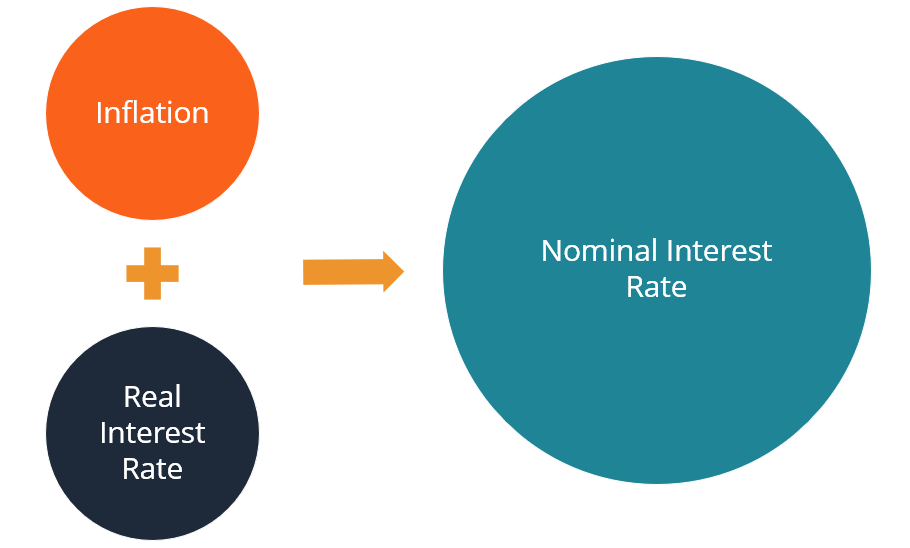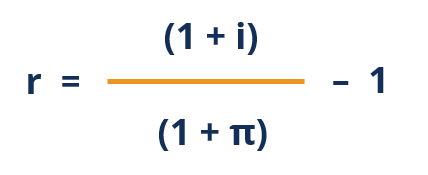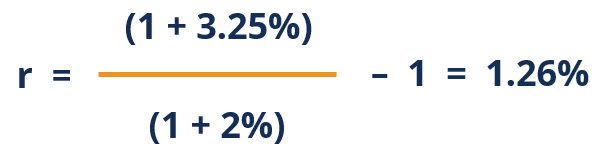# Fisher Equation

The nominal interest rate is equal to the sum of the real interest rate and inflation

## What is the Fisher Equation?

The Fisher equation is a concept in economics that determines the relationship between nominal and real interest rates under the effect of the inflation. The equation states that the nominal interest rate is equal to the sum of the real interest rate and inflation.

The Fisher equation describes a situation where investors or lenders ask for an additional reward to compensate for losses in purchasing power due to higher inflation.The concept is widely used in the fields of finance and economics. It is frequently used in calculating returns on investments or in predicting the behavior of nominal and real interest rates. One example is when an investor wants to determine the actual (real) interest rate earned on an investment after accounting for the effect of inflation.

One particular significance of the Fisher equation is related to monetary policy. The equation reveals that the monetary policy moves the inflation and nominal interest rate together in the same direction. On the other hand, the monetary policy generally does not affect the real interest rate.

American economist Irving Fisher proposed the equation.

### Fisher Equation Formula

Mathematically, the Fisher equation is generally expressed through the following formula:

##### (1 + i)  = (1 + r) (1 + π)

Where:

i – the nominal interest rate

r – the real interest rate

π – the inflation rate

However, we can also see the approximate version of the previous formula:

### Fisher Equation Example

Suppose Sam owns an investment portfolio. Last year, the portfolio earned a return of 3.25%. However, last year’s inflation was around 2%.

Sam wants to determine the real interest rate he earned from the portfolio. In order to find the real interest rate, we should use the Fisher equation. The equation states that:

##### (1 + i)  = (1 + r) (1 + π)

We can rearrange the equation to find real interest rate:Therefore, the real interest rate of the investment portfolio equals:The real interest rate that Sam’s investment portfolio earned last year after accounting for the inflation effect is 1.26%.

Thank you for reading CFI’s explanation of the Fisher equation. CFI offers the Financial Modeling & Valuation Analyst (FMVA)™ certification program for those looking to take their careers to the next level. To keep learning and advancing your career, the following resources will be helpful:

• Effective Annual Interest Rate
• Floating Interest Rate
• Normative Economics

### Financial Analyst Certification

Become a certified Financial Modeling and Valuation Analyst (FMVA)® by completing CFI’s online financial modeling classes!

### Financial Analyst Training

Get world-class financial training with CFI’s online certified financial analyst training program!

Gain the confidence you need to move up the ladder in a high powered corporate finance career path.

Learn financial modeling and valuation in Excel the easy way, with step-by-step training.